Home   |   My Cart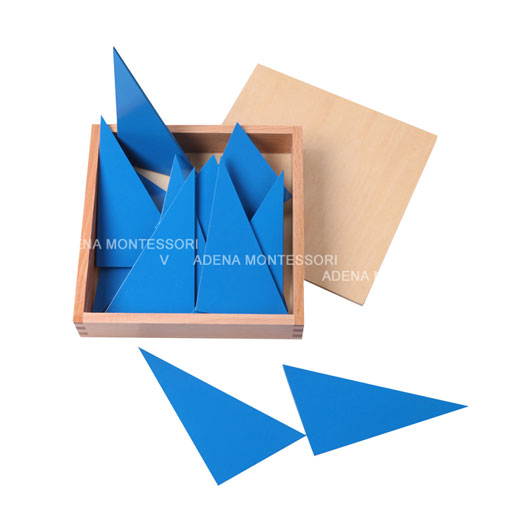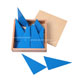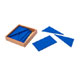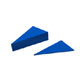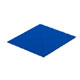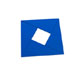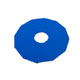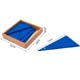A150
Box of Blue Triangles

Unit Price: \$38.15

Discount: 51% off

Savings: \$59.31

Market Price : \$78.00

In Stock.

Qty:

Twelve blue right-angled scalene triangles with no black lines. The angles measure 30, 60, and 90 degrees.

Presentation

1. Isolate one triangle.
2. Ask the child to identify each of the angles, and the biggest and smallest angles.
3. This angle which is neither smallest nor biggest we can call the medium angle.
4. The child names each angle: smallest angle, medium angle, biggest angle.
5. First star: Let's unite all the triangles by their smallest angle.
6. The directress positions a few and allows the child to continue.
7. How many points does this star have? Twelve.
8. With all of the triangles at our disposal, we can make only one star with twelve points.
9. Second star: Let's unite all the triangles by the medium angles.
10. How many points does this star have? Six.
11. Try to make another star with the triangles that are left.
12. With all the triangles at our disposal, we can make two stars with six points.
13. Third star: Let's unite all of the triangles by the largest angle.
14. How many points does this star have? Four.
15. This symbol is very famous; it is the star of Saint Brigid, the patron saint of Ireland.
16. Try to make another star like this.
17. With all the triangles at our disposal, we can make three stars with four points.

Purpose

Use of the triangle as a constructor to indirectly demonstrate the following:

• 30o x 12 triangles = 360o
• 60o x 6 triangles = 360o
• 90o x 4 triangles = 360o
• 360o / 30o = 12 triangles
• 360o / 60o = 6 triangles
• 360o / 90o = 4 triangles
• 360o / 12 triangles = 30o
• 360o / 6 triangles = 60o
• 360o / 4 triangles = 90o
Purchasing this will earn you 19 rewards points!
Dimensions: box size:17.5*17.5*4.5cm,packing size:19*19*5.5cm

Weight: 0.5kg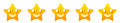-------------------------------------------------------------------
1 Point 2 Points 3 Points 4 Points 5 Points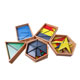A093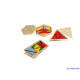A094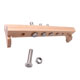A280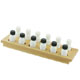A120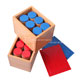A110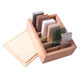A130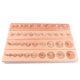A020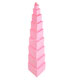A040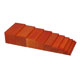A061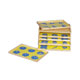A100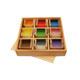A072